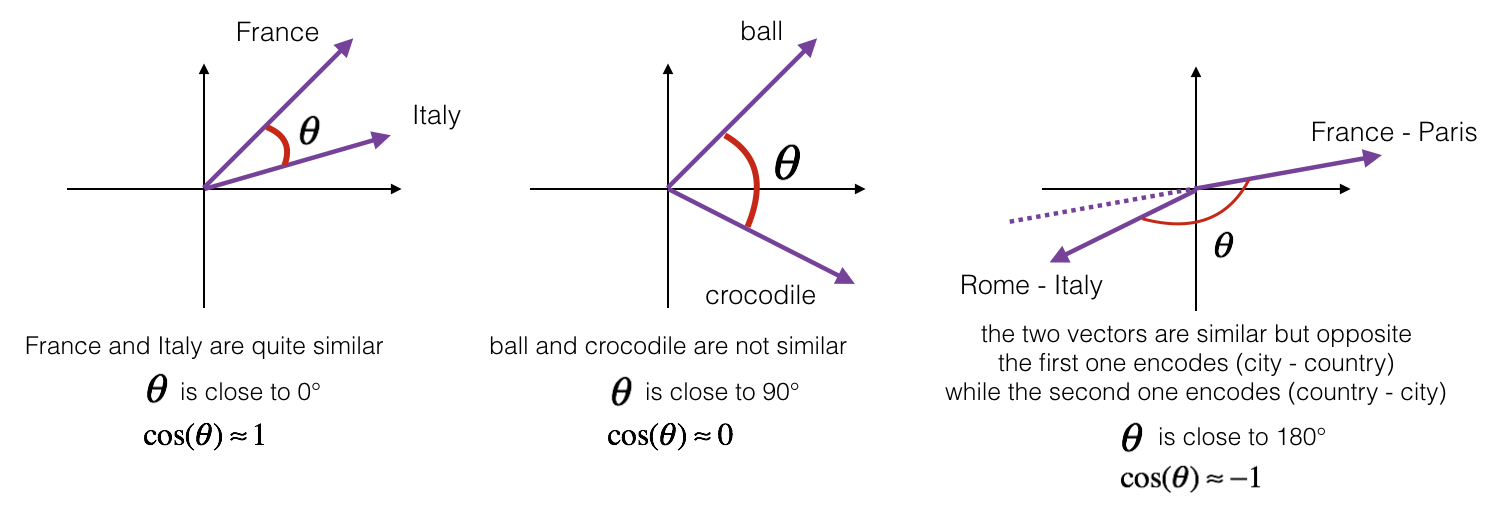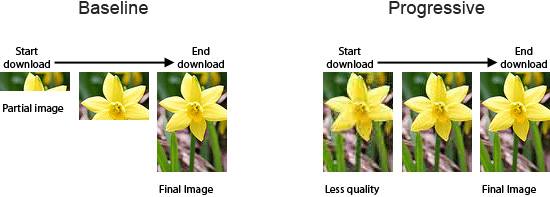# 余弦定理与文本相似度钱魏Way · · 3 次浏览

## 什么是余弦定理

$$\cos {A} =\frac{b^2+c^2-a^2}{2bc}$$

$$\cos {A} = \frac{<b,c>}{|b||c|}$$

• x_1,x_2,…,x_64000
• y_1,y_2,…,y_64000

$$\cos {\theta } = \frac{x_1y_1+x_2y_2+…+x_{64000}y_{64000}}{\sqrt{x_1^2+x_2^2+…+x_{64000}^2}\cdot \sqrt{y_1^2+y_2^2+…+y_{64000}^2}}$$## 计算文本相似度的大致流程

• A：我喜欢看电视，不喜欢看电影。
• B：我不喜欢看电视，也不喜欢看电影。

• A：我/喜欢/看/电视，不/喜欢/看/电影。
• B：我/不/喜欢/看/电视，也/不/喜欢/看/电影。

• A：我 1，喜欢 2，看 2，电视 1，电影 1，不 1，也 0。
• B：我 1，喜欢 2，看 2，电视 1，电影 1，不 2，也 1。

• A：[1, 2, 2, 1, 1, 1, 0]
• B：[1, 2, 2, 1, 1, 2, 1]

• 实际使用中文本长度可能过长，如果采用分词，复杂度较高，可以采用TF-IDF的方式找出文章若干个关键词（比如20个），再进行比较。
• 文章的长度可能不一致，所以关键词词频率可以使用相对词频率。
• 由于计算中打乱了关键词出现的顺序，所以即使夹角余弦的值为1，也有可能文本并不重复。比如：
• A：我喜欢看电视，不喜欢看电影。
• B：我不喜欢看电视，喜欢看电影。

## 使用Python进行文本相似度计算

#!/usr/bin/env python
# -*- coding: utf-8 -*-
from __future__ import division
import jieba.analyse
from math import sqrt

class Similarity():
def __init__(self, target1, target2, topK=10):
self.target1 = target1
self.target2 = target2
self.topK = topK

def vector(self):
self.vdict1 = {}
self.vdict2 = {}
top_keywords1 = jieba.analyse.extract_tags(self.target1, topK=self.topK, withWeight=True)
top_keywords2 = jieba.analyse.extract_tags(self.target2, topK=self.topK, withWeight=True)
for k, v in top_keywords1:
self.vdict1[k] = v
for k, v in top_keywords2:
self.vdict2[k] = v

def mix(self):
for key in self.vdict1:
self.vdict2[key] = self.vdict2.get(key, 0)
for key in self.vdict2:
self.vdict1[key] = self.vdict1.get(key, 0)

def mapminmax(vdict):
"""计算相对词频"""
_min = min(vdict.values())
_max = max(vdict.values())
_mid = _max - _min
#print _min, _max, _mid
for key in vdict:
vdict[key] = (vdict[key] - _min)/_mid
return vdict

self.vdict1 = mapminmax(self.vdict1)
self.vdict2 = mapminmax(self.vdict2)

def similar(self):
self.vector()
self.mix()
sum = 0
for key in self.vdict1:
sum += self.vdict1[key] * self.vdict2[key]
A = sqrt(reduce(lambda x,y: x+y, map(lambda x: x*x, self.vdict1.values())))
B = sqrt(reduce(lambda x,y: x+y, map(lambda x: x*x, self.vdict2.values())))
return sum/(A*B)

if __name__ == '__main__':
t1 = '''余弦定理和新闻的分类似乎是两件八杆子打不着的事，但是它们确有紧密的联系。具体说，新闻的分类很大程度上依靠余弦定理。Google 的新闻是自动分类和整理的。所谓新闻的分类无非是要把相似的新闻放到一类中。计算机其实读不懂新闻，它只能快速计算。这就要求我们设计一个算法来算出任意两篇新闻的相似性。为了做到这一点，我们需要想办法用一组数字来描述一篇新闻。我们来看看怎样找一组数字，或者说一个向量来描述一篇新闻。回忆一下我们在“如何度量网页相关性”一文中介绍的TF/IDF 的概念。对于一篇新闻中的所有实词，我们可以计算出它们的单文本词汇频率/逆文本频率值（TF/IDF)。不难想象，和新闻主题有关的那些实词频率高，TF/IDF 值很大。我们按照这些实词在词汇表的位置对它们的 TF/IDF 值排序。比如，词汇表有六万四千个词，分别为'''
t2 = '''新闻分类——“计算机的本质上只能做快速运算，为了让计算机能够“算”新闻”(而不是读新闻)，就要求我们先把文字的新闻变成一组可计算的数字，然后再设计一个算法来算出任何两篇新闻的相似性。“——具体做法就是算出新闻中每个词的TF-IDF值，然后按照词汇表排成一个向量，我们就可以对这个向量进行运算了，那么如何度量两个向量？——向量的夹角越小，那么我们就认为它们更相似，而长度因为字数的不同并没有太大的意义。——如何计算夹角，那就用到了余弦定理（公式略）。——如何建立新闻类别的特征向量，有两种方法，手工和自动生成。至于自动分类的方法，书本上有介绍，我这里就略过了。很巧妙，但是我的篇幅肯定是放不下的。除余弦定理之外，还可以用矩阵的方法对文本进行分类，但这种方法需要迭代很多次，对每个新闻都要两两计算，但是在数学上有一个十分巧妙的方法——奇异值分解(SVD)。奇异值分解，就是把上面这样的大矩阵，分解为三个小矩阵的相乘。这三个小矩阵都有其物理含义。这种方法能够快速处理超大规模的文本分类，但是结果略显粗陋，如果两种方法一前一后结合使用，既能节省时间，又提高了精确性。'''
topK = 10
s = Similarity(t1, t2, topK)
print s.similar()##### 使用渐进式JPEG来提升用户体验##### 网站开发图片格式对比与选择